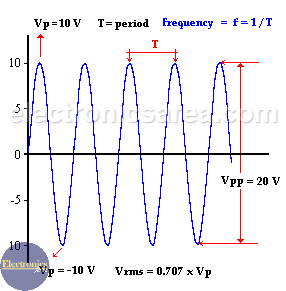# What is Alternating Current?

The difference between an alternating current and a continuous current is that the continuous current flows only on one direction. The alternating current circulates first in one direction and then on the opposite one, repeating this process continuously.

This is the kind of electric current that we have in our houses to use the TV, the stereo, the washing machine, the refrigerator, etc..

The following picture shows the magnitude variation of a voltage (which is also alternating). First it goes up and then down and it gives us a sine waveform. (it behaves the same way as the current).

This voltage (and current) varies continuously, and we can use the formula: V = Vp x Sin(Θ) to know the voltage at a specific time. Note: Θ = wt = 2πft, where:

• π = 3.1416
• f = frequency
• t = time

The Vp is the maximum value of the wave and Θ is the angular distance that is measured in degrees. See the diagram.Looking at the last picture, we see a periodic sine wave (the wave form repeats continuously) and a complete cycle of this sine wave has 360 degrees. We can find the voltage magnitude at any time with the above formula.

For each angular distance Θ there is a different voltage. Where in some cases it’s positive and in others negative (when it reverses its polarity.)

## Properties of Alternating Current

• Frequency: (f) The number of cycles that a signal (voltage or current) has in one second. The frequency unit is: Hertz
• Period: (T) The interval of time needed for a signal to be repeated. The Period has the formula: T = 1/f or the period (T) is the inverse of the frequency (f).
• Peak-to-peak Alternating Current voltage (Vpp)
Looking at the picture we see that there is a maximum and a minimum voltage.

The difference between these two alternating current voltage values is called peak-to-peak voltage (Vpp) and is twice the peak Voltage (Vp) (see the picture).

•  RMS value. RMS voltage (Vrms): You can get the RMS value of an Alternating Current voltage using the formula: Vrms = 0,707 x Vp. This is the voltage value we get from a VOM / Multimeter.

Now, here’s something to think about.

When we use a VOM to test the AC voltage in our houses, we find that this voltage will be near 110 or 220 volts (depends on the country we live). This is an RMS voltage. What will the peak voltage (Vp) of this signal be?

Using the formula above: Vp = Vrms / 0,707.

case 1: Vrms = 110 V, then Vp = 110/0,707 = 155.6 Volts.
case 2: Vrms = 220 V, then Vp = 220/0,707 = 311.17 Volts.

•
•
• 4
•
•
•
•
•
4
Shares
•
4
Shares
•
•
• 4
•
•
•
•